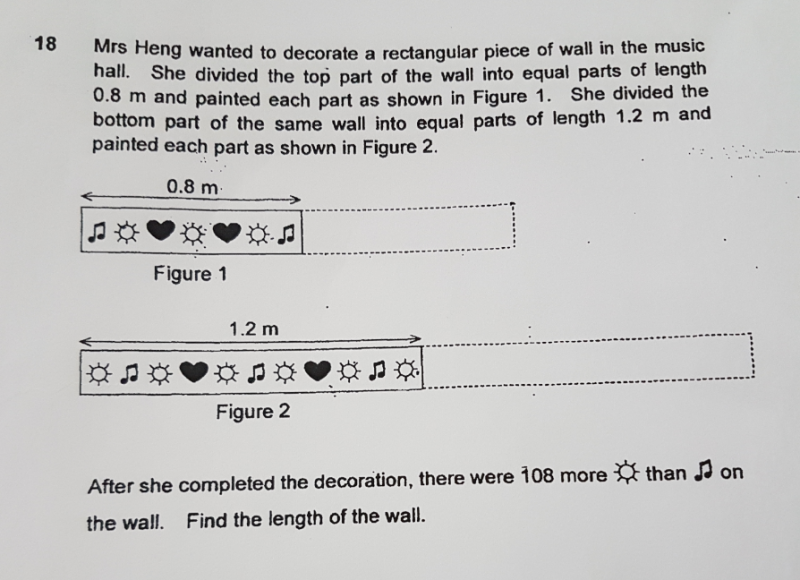# QuestionHi, we need help with this qn! Tq:)

This is a Quantity Each Product question.

LCM

0.8 × 3 = 2.4      sun – music = (3 -2)  = 1

1.2 × 2 = 2.4      sun – music = (6  – 3) = 3

 Quantity Each Product F1 3u 1 3u F2 2u 3 6u 9u

9u = 108

1u = 108 ÷ 9 = 12

2u = 24

24 × 1.2 = 28.8 m

0 Replies 0 Likes